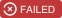# Polylearn

A library for factorization machines and polynomial networks for classification and regression in Python.
Alternatives To Polylearn
3 days ago4October 28, 2020136apache-2.0Python
Competitive Recsys475
2 years ago
A collection of resources for Recommender Systems (RecSys)
Pytorch Fm268
3 years ago8January 18, 20203mitPython
Factorization Machine models in PyTorch
Recommend System Tf2.0262
2 years ago3Python

Polylearn207
3 years ago7bsd-2-clausePython
A library for factorization machines and polynomial networks for classification and regression in Python.
Tensorflow In Practice33
4 years agoPython
Code based in TensorFlow
Lightctr32
4 years ago2Python
LightCTR is a tensorflow 2.0 based, extensible toolbox for building CTR/CVR predicting models.
Dl_ctr25
5 years ago1Jupyter Notebook

Fscnmf16
a year agogpl-3.0Python
An implementation of "Fusing Structure and Content via Non-negative Matrix Factorization for Embedding Information Networks".
Tensorflow2_model_zoo10
4 years ago2Jupyter Notebook
explore tensorflow 2
Alternatives To Polylearn
Select To Compare

Alternative Project Comparisons

# polylearn

A library for factorization machines and polynomial networks for classification and regression in Python.

Github repository.Factorization machines and polynomial networks are machine learning models that can capture feature interaction (co-occurrence) through polynomial terms. Because feature interactions can be very sparse, it's common to use low rank, factorized representations; this way, we can learn weights even for feature co-occurrences that haven't been observed at training time.

Factorization machines are popular for recommender systems, as they are a generalization of matrix completion models.

This package provides:

• coordinate descent algorithm for fitting factorization machines of degree 2 or 3,
• coordinate descent algorithm for fitting polynomial networks of arbitrary degree,
• scikit-learn-compatible API,
• Cython implementations for computationally intensive parts.

## Installation

Binary packages are not yet available.

The development version of polylearn can be installed from its git repository. In this case it is assumed that you have a working C++ compiler.

1. Obtain the sources by:

```git clone https://github.com/scikit-learn-contrib/polylearn.git
```

1. Install the dependencies:

```# via pip

pip install numpy scipy scikit-learn nose
pip install sklearn-contrib-lightning

# via conda

conda install numpy scipy scikit-learn nose
conda install -c conda-forge sklearn-contrib-lightning
```
2. Build and install polylearn:

```cd polylearn
python setup.py build
sudo python setup.py install
```

## References

The solvers implemented are introduced in . Factorization machines are introduced in  and polynomial networks in .

  Mathieu Blondel, Masakazu Ishihata, Akinori Fujino, Naonori Ueda. Polynomial Networks and Factorization Machines: New Insights and Efficient Training Algorithms. In: Proc. of ICML 2016. [PDF]
  Steffen Rendle. Factorization machines. In: Proc. of IEEE ICDM 2010. [PDF]
  Roi Livni, Shai Shalev-Shwartz, Ohad Shamir. On the computational efficiency of training neural networks. In: Proc. of NIPS 2014. [arXiv]